1. Analysis of single SNPs

A polymorphism occurs when different individuals have many genetic variants at the same location (loci) of their genomes. Every possible variant is called allele, and if only one nucleotide has changed, the variant is named SNP (Single Nucleotide Polymorphism). In this case, we usually have two possibilities for each loci, for example changing T by C (denoted T→C). Then we say this loci is biallelic. Every individual carries two alleles of every loci, one for each of the 22 autosomic chromosomes inherited independently from his parents. A genotype is an observed couple of individual alleles for some loci. In the former example, there are three potential genotypes: T/T, T/C and C/C. If the two alleles are identical (T/T or C/C) we say the individual is homozygous. Otherwise, the individual will be heterozygous. We can establish the variant genotype as the less frequent, but it can differ amog different populations.

1.1 Descriptive analysis

The statistical analysis of a polymorphism is based on estimating the population prevalence of each allele, and by doing it for each possible genotype we can then estimate the genotype and allele frequencies. To estimate the genotype frequencies () we calculate the observed rate of genotypes. Let be N the sample size, then: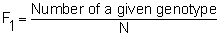To see an application, consider the test sample data included in the SNPstats software (dataset 1). This dataset has information about 5 biallelic locus for 706 individuals. We choose one specific SNP (SNP2) and the software performs the genotype frequencies (proportion") for all the subjects and separately for every status subset, as shown in Figure 1:

 Genotype frequencies (n=706) All subjects STATUS=0-Control STATUS=1-Case Genotype Count Proportion Count Proportion Count Proportion T/T 255 0.4 123 0.41 132 0.4 T/C 264 0.42 126 0.42 138 0.41 C/C 116 0.18 52 0.17 64 0.19 NA 71 --- 28 --- 43 ---

Figure 1. Note that the proportions are calculated considering the 635 individuals with genotype information.

To calculate allele frequencies (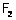) we double the sample chromosomes and count every allele rate: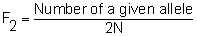The results of applying this formula to SNP2 are shown in the next table:

 Allele frequencies (n=635) All subjects STATUS=0-Control STATUS=1-Case Allele Count Proportion Count Proportion Count Proportion T 774 0.61 372 0.62 402 0.6 C 496 0.39 230 0.38 266 0.4

Figure 2. Allele frequencies.

1.1.2 Hardy-Weinberg Equilibrium

First thing we have to do before beginning an association analysis between genetic polymorphisms and diseases is a test for Hardy-Weinberg equilibrium. This is useful to assess independence between the alleles inherited from the parents. The statistic test

HW=compares the observed allele frequency with the expected one under the assumption of independence, and has a chi-square distribution with one degree of freedom.

We consider again the polymorphic biallelic loci SNP2 with a sample of size N=706 (71 missings) and two possible nucleotides, T and C, with probabilities p=0.61 and q=0.39 respectively. The expected frequencies for each genotype are N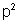(=236,28) for T/T, 2Npq (=302.13) for T/C and N(=96.58) for C/C which are compared with the observed genotype frequencies shown in Figure 1.

The next table shows the p-values calculated via an exact test:

 Exact test for Hardy-Weinberg equilibrium (n=635) N11 N12 N22 N1 N2 P-value All subjects 255 264 116 774 496 0.0015 STATUS=0-Control 123 126 52 372 230 0.051 STATUS=1-Case 132 138 64 402 266 0.012

Figure 3. Hardy-Weinberg equilibrium.

If the deviation was significative, first check the genotyping method to discard a bias. At last the individuals could be dependents due to the sampler method.

1.2 Analysis of association between polymorphisms and disease

The statistic point of view lead us to describe a polymorphism like a categorical variable with one level for each possible genotype. The reference category often is the homozygous form.

To assess association between one polymorphism and disease we make the contingency table and then apply a chi-square test. The estimation of the OR (odds ratio) for each genotype respect to the reference genotype will give us a notion about the measure of the association.

In case we need to adjust the model by confounders variables, it would rather use logistic regression models due to their versatility. These models easily allow to assess interaction between the polymorphism and the other factors.

Let us to describe the logistic regression model. Let be p the case probability, G the categorical variable with the polymorphisms codified and Z the variables to adjust the model. The next equation defines the logistic model:This equation involves three parameters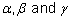that must be estimated.

Now let be G a SNP with a variant allele C which modifies the risk of be case. Like mentioned above, every individual genotype is formed by two alleles. Now, the risk of every genotype depends on the number of C copies carried (one or two). According to the number of copies needed in order to modify the risk, there are five inheritance models we can define:

Co-dominant model: Is the most general model and it allows every genotype to give a different and non additive risk. This model compares heterozygous T/C (He) and homozygous for the variant allele C/C (Va) genotypes to the homozygous for the most frequent allele T/T: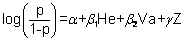This model estimates two ORs, one for He and one for Va.

Dominant model: A single copy of C is enough to modify the risk, then heterozygous and homozygous genotypes have the same risk. We could compare a combination of these two possible genotypes T/C+C/C (Do) to the homozygous T/T.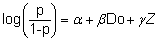Recessive model: Two copies of C are necessary to change the risk. Hence, T/C and T/T genotypes have the same effect. A combination of both T/T+T/C (Re) is compared to the variant allele homozygous genotype C/COver-dominant model: Heterozigous are compared to a pool of both allele homozygous, it is T/C (He) is compared versus T/T+C/C.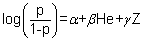Additive model: Each copy of C modifies the risk in an additive form, it is the homozygous C/C have double risk than heterozygous T/C. Now, compare a combination of the two genotypes with weights 2 and 1 respectively 2C/C+T/C (Ad), to T/T: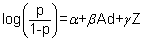Look below the models performed for SNP2 and the ORs for each comparison:

 SNP association with disease (crude analysis) Model Genotype STATUS=0-Control STATUS=1-Case OR (CI 95%) P-value AIC Codominant T/T 123 (40.9%) 132 (39.5%) 1.00 0.82 884.2 T/C 126 (41.9%) 138 (41.3%) 1.02 (0.72-1.44) C/C 52 (17.3%) 64 (19.2%) 1.15 (0.74-1.78) Dominant T/T 123 (40.9%) 132 (39.5%) 1.00 0.73 882.5 T/C-C/C 178 (59.1%) 202 (60.5%) 1.06 (0.77-1.45) Recessive T/T-T/C 249 (82.7%) 270 (80.8%) 1.00 0.54 882.2 C/C 52 (17.3%) 64 (19.2%) 1.14 (0.76-1.70) Overdominant T/T-C/C 175 (58.1%) 196 (58.7%) 1.00 0.89 882.6 T/C 126 (41.9%) 138 (41.3%) 0.98 (0.71-1.34) Additive --- --- --- 1.06 (0.86-1.31) 0.58 882.3

Figure 4. The last two columns are useful for choose the best model.

1.2.1 Choose the best model

Given a SNP, a criterion is necessary to decide the best inheritance model. To compare every model to the most general model (the co-dominant) we can select the likelihood ratio test (LRT). The likelihood ratio test is a statistical test of the goodness-of-fit between two models: we compare a relatively more complex model to a simpler model to asses if it fits a dataset better.

The LRT compares the likelihood scores of the two models:

LR = 2*(lnL1-lnL2)

The statistic follows a chi-square distribution with degrees of freedom equal to the number of additional parameters in the more complex model. Even so, sometimes this test is not enough to discard models, and criteria like the Akaike information (AIC) could be useful to choose the inheritance model that best fits the data. It is, the model with the less akaike information value, that corresponds to minimize the expected entropy :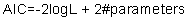Figure 4. shows the p-value of the likelihood ratio test for SNP2 and the AIC value. The inheritance model with less AIC is the recessive.

1.3 Analysis of interactions with covariates

For the interaction models a new term is added: the product between the genotype and an environment variable: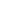or between two genotype variables: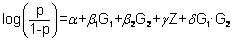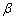-coefficients allow us to describe the association between each polymorphisms and the disease by way of calculate the ORs. Therefore, the corresponding 95% confidence intervals are also computable.

Let be Z the categorical covariate SEX of the sample data. The next tables show ORs for every SNP2s variant relative to the most frequent, calculated for each covariate level (male/female):

 Corner interaction table (crude analysis) Female Male STATUS=0-Control STATUS=1-Case OR (95% CI) STATUS=0-Control STATUS=1-Case OR (95% CI) T/T 59 50 1.00 64 82 1.51 (0.92-2.49) T/C 57 55 1.14 (0.67-1.93) 69 83 1.42 (0.87-2.33) C/C 22 21 1.13 (0.56-2.28) 30 43 1.69 (0.93-3.08)

Figure 5.

First of the next two tables (Figure 6.) shows the ORs classifying first by the SNP, and then by SEX. The second table, first separates by SNP and then by SEX:

SEX within SNP2 (crude analysis)

T/T

 STATUS=0-Control STATUS=1-Case OR (95% CI) Female 59 50 1.00 Male 64 82 1.51 (0.92-2.49)

T/C

 STATUS=0-Control STATUS=1-Case OR (95% CI) Female 57 55 1.00 Male 69 83 1.25 (0.76-.03)

C/C

 STATUS=0-Control STATUS=1-Case OR (95% CI) Female 22 21 1.00 Male 30 43 1.50 (0.70-3.21)

Interaction p-value: 0.84 Trend test: 0.87

Figure 6. Two p-values are provided, one for the interaction and another for the trend.

SNP2 within SEX (crude analysis)

Female

 STATUS=0-Control STATUS=1-Case OR (95% CI) T/T 59 50 1.00 T/C 57 55 1.14 (0.67-1.93) C/C 22 21 1.13 (0.56-2.28)

Male

 STATUS=0-Control STATUS=1-Case OR (95% CI) T/T 64 82 1.00 T/C 69 83 0.94 (0.59-1.48) C/C 30 43 1.12 (0.63-1.98)

Interaction p-value: 0.84 Trend test: 0.84

Figure 7.

2. Analysis of multiple SNPs

In many situations the causal polymorphism is unknown. In order to locate it, more than one polymorphism are taking into account to be analyzed.

The statistic correlation observable between different polymorphisms closer located in the same chromosome, is called Linkage disequilibrium. This association has its origin in the meiosis, the process of cell division which occurs in the maturation of sex cells. At the end of the process therell be two gametes, each gamete with one copy of each couple of chromosomes. Take notice these chromosomes wont be identical to the parental chromosomes due to the recombination (fragments of different copies of the same couple of chromosomes are interchanged during the meiosis). Thereby, the chromosomes inherited by the offspring will be a combination between mother and father chromosomes.

Even so, the probability of have a recombination between closer locus are very low, and usually these locus are transmitted in block. So that, the polymorphisms next to the causal will be in association with the disease too, and analyze sets of locus could be very useful for locate the true causal polymorphism.

The set of polymorphisms transmitted together in every resultant chromosome is denominated haplotype. Given the sample genotypes, every individual has two possible haplotypes, one per chromosome. Undesirably, usually this genotypes are given with non chromosomical location, because in many cases sophisticated laboratory techniques are required to. Due to this lack of information, when an individual has at last two heterozygous locus his couple of haplotypes is unknown and in practice, estimation methods like the EM algorithm or Markov Chain Monte Carlo methods are used.

To determine the linkage disequilibrium we calculate the D statistic, it is the deviation between the expected haplotype frequency (under the assumption of no association) and the observed frequency. Let beand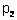the probabilities of two alleles, and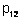the observed probability of the couple. Then,

D=-SNPstats also offers the D' statistic that is equal to D scaled in [-1,1] range.

The correlation coefficient between alleles is provided too.

Look at the next table to see the results for two SNPs (SNP1 and SNP2) given by SNPstats:

 D statistic D' statistic r statistic Snp1 Snp2 Snp1 Snp2 Snp1 Snp2 Snp1 . 0.2157 Snp1 . 0.9814 Snp1 . 0.9206 Snp2 . . Snp2 . . Snp2 . .

Figure 8.

2.2 Analysis of haplotypes

Some individuals have uncertain haplotypes due to the lack of chromosomical information mentioned above. Hence the count of sample haplotypes isnt straightforward. Moreover, missing values in the data increase this uncertainty.

There are many different methods to estimate the Haplotype frequency. One of the most commonly used is the two-stage iterative method named EM algorithm (Expectation Maximization algorithm).

First, initial values for the haplotype frequencies are given. Then, the E pass consists in recalculate the expected genotype frequency for the genotypes with uncertainty haplotypes (under Hardy Weinberg equilibrium) using the haplotypes frequency . Using the recalculated genotype frequencies, the M pass calculates every haplotype frequency. It is, count the compatibles haplotypes for every genotype. At last, the algorithm converges desirably to the haplotype frequencies. It could be advisable to repeat the method with different start point to avoid local maximums.

The haplotype frequency estimated via the EM Algorithm for two SNPs (SNP1 and SNP2) is shown in the next table:

 Haplotype frequencies estimation SNP1 SNP2 Total group.0.Control group.1.Case CumFreq 1 C T 0.6062 0.6169 0.5965 0.6062 2 G C 0.3556 0.3614 0.3504 0.9618 3 C C 0.0341 0.02 0.0469 0.9959 4 G T 0.0041 0.0017 0.0062 1

Figure 9. Haplotype frequencies are shown for each level of the status variable. The cumulative frequency is useful to decide which haplotypes are rare.

2.2.1 Analysis of association between haplotypes and disease

If theres no uncertainty, the individual has his own couple of haplotypes. The association between haplotypes and disease can be analyzed via logistic regression. A chromosome analysis is usually done instead of treating individuals. The sample is duplicated and then each individual is doubly represented with his two haplotypes. The risk for every haplotype will be compared respect to the reference category, it is the most frequent haplotype.

If there is uncertainty, methods like the EM algorithm lead us to have a rebuilt haplotype sample. The uncertain individuals contribute with more than two haplotypes, it is with the haplotypes compatibles with his genotype. In this case, every haplotype take a different weight in the logistic regression model.

Analyzing the association between haplotypes related to SNP1 and SNP2, and disease, SNPstats the next table:

 Haplotype association with disease (crude analysis) SNP1 SNP2 Freq OR (95% CI) P-value 1 C T 0.6062 1.00 --- 2 G C 0.3556 0.99 (0.80 - 1.23) 0.94 3 C C 0.0342 2.41 (1.22 - 4.75) 0.011 rare * * 0.0041 3.78 (0.42 - 34.24) 0.24

Figure 10. The significative OR is red written